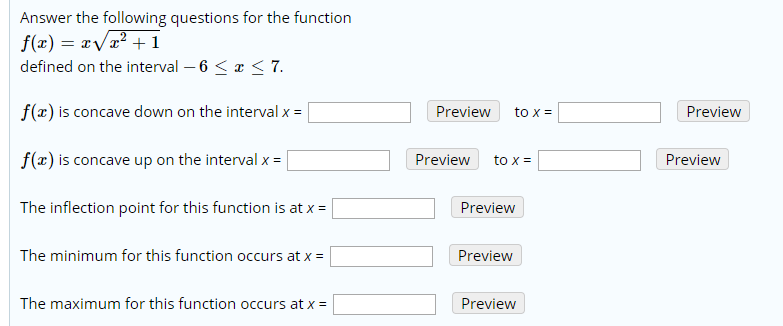# Answer the following questions for the functionf(x) = x/x2 +1defined on the interval 6 < x < 7f(x) is concave down on the interval x =PreviewPreviewto xf(x) is concave up on the interval xPreviewPreviewto xThe inflection point for this function is at x =PreviewThe minimum for this function occurs at xPreviewThe maximum for this function occurs at xPreview

Question
1 viewshelp_outlineImage TranscriptioncloseAnswer the following questions for the function f(x) = x/x2 +1 defined on the interval 6 < x < 7 f(x) is concave down on the interval x = Preview Preview to x f(x) is concave up on the interval x Preview Preview to x The inflection point for this function is at x = Preview The minimum for this function occurs at x Preview The maximum for this function occurs at x Preview fullscreen
check_circle

Step 1

First draw the graph of f(x) by using the table:

Step 2

Graph

Step 3

The given function is continuous and differential and defined on the given interval (-6,7).

The graph of the function is said to be Concave downw...

### Want to see the full answer?

See Solution

#### Want to see this answer and more?

Solutions are written by subject experts who are available 24/7. Questions are typically answered within 1 hour.*

See Solution
*Response times may vary by subject and question.
Tagged in

### Calculus